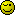How do you add and subtract \$xx.xx money values using php?

I’ve tried using the

"\$addition = 2 + 4; "

type code but it doesn’t seem to work with decimal points.

it works with decimal points. Are you trying to add dollar amounts with the dollar sign attached?

``````
\$decimal = number_format(4 + 2, 2);

``````

so?

\$decimal = number_format(4.2 + 2.2);

no

``````number_format(4.2 + 2.2, 2)
``````

Why is there a ,2 there?

It’s the number of digits after the decimal point [2 = X.xx, 4 = X.xxxx…etc]

look up number_format on the php website!@@#%@#You would be better off doing that only when displaying the value though. There is no need to do it when adding the number together.

Is it possible to take the results of different recordsets and add them this way? Maybe like this…

``````\$recordset1 = rs1Result
\$recordset2 = rs2Result
\$recordset3 = rs3Result

\$total = number_format(\$recordset1 + \$recordset2 + \$recordset3, 2);
``````

No need? What difference does it make? Easier to do it like this. That way you can separate the programming area and the designing code.

• Tomer

you should be doing that in the SQL statement, not your PHP code.

In most cases you do a calculation on the various amounts multiple times before any amount is displayed for the user, if it is even displayed.

In these cases it would not be necessary to use the function, it would just add overhead to the script.

We dont want lukkyjay to believe he is required to use that function each time he sums decimal numbers.

Can you say something like

“Add all the numbers in column”

If you want to sum together all the numbers in the table for a specific column etc you can use SUM().

Examples:
SELECT SUM(amount) as total FROM table
SELECT SUM(amount) as total FROM table WHERE userid=1
SELECT year, SUM(amount) as total FROM table WHERE userid=1 GROUP BY year

You should read up on the math functions available for mysql (or the database type you use)

sure.

``````
\$sql = "SELECT SUM(columnname) FROM tablename";
\$query = mysql_query(\$sql);
\$total = mysql_result(\$sql, 0);

``````

edit: looks like reddevil beat me to itIs there something I’m missing?

``````<?php
session_start();
include("constring.php");
?>

<?php
\$sql = "SELECT SUM(amount) FROM income";
\$query = mysql_query(\$sql);
\$total = mysql_result(\$sql, 0);

echo "\$total";
?>
``````

yes, you’re not telling us what the problem is.Haha! I’m getting this error sorry:

Warning: mysql_result(): supplied argument is not a valid MySQL result resource in /home/url/public_html/ledger/index.php on line 9

``````\$total = mysql_result(\$query, 0);